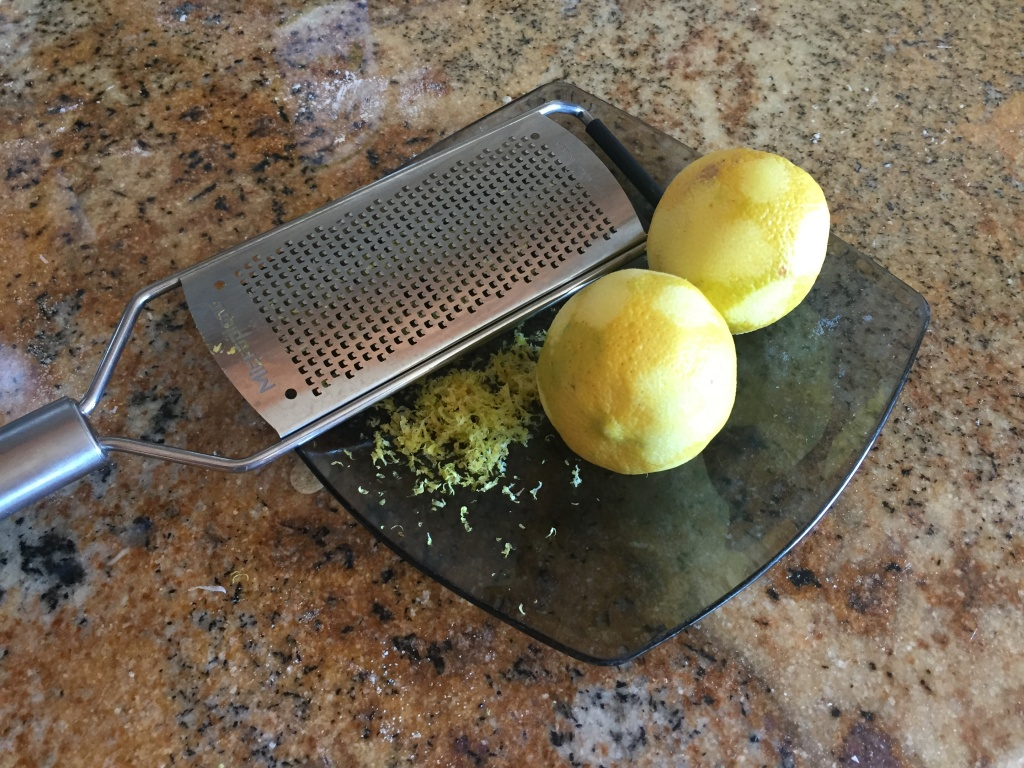# Lemon Zest and the Surface Area of a SphereI was recently asked to bring a cake to a reception on very short notice. Luckily, I found this recipe for Olive Oil Cake  by Marcella Hazan, for which I had all the ingredients. I had two small lemons left in the fridge, and I conveniently assumed that the zest of both of them will be equivalent to the “zest of one lemon” required by the recipe.

Was I right?  Just out of curiosity, I asked myself:

What would be the size of the hypothetical one lemon which would yield the same amount of zest as my two small lemons?

Would this be a gargantuan lemon of mythical size, or just a regular looking fruit? This was easy enough to determine, since my two small lemons were nearly spherical, with diameter of about 2 inches:The lemon zest layer is so thin, that its amount can be measured by the surface area of the lemon times its thickness.

Using the formula for the surface area of a sphere of radius r,

Surface Area =4πr²,

and the fact that our lemons have a radius of about an inch, we have that the surface area of each lemon is about 4π square inches. So the surface area of the two small lemons is 8π square inches. How large a single lemon would have to be in order to have surface area of 8π square inches? Its radius, R, is given by this equation:

8π = 4πSo R=√2≈1.4 inches. That’s not that big! At just 2.8 inches diameter, this hypothetical lemon would be well inside the range of lemons supplied by Sunkist growers. So it was a good call to replace this lemon with two little ones!

SaveSave

SaveSave